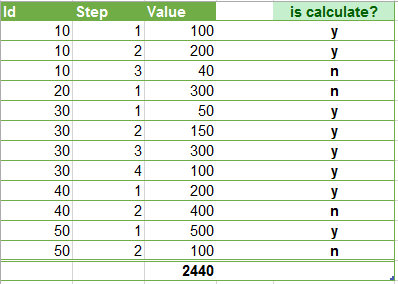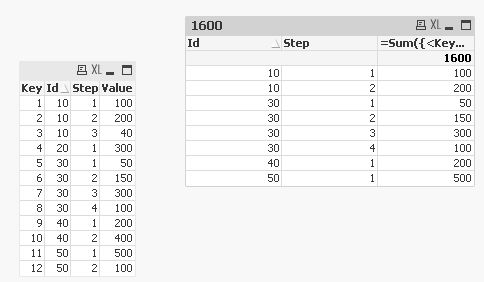# QlikView App Development

Discussion Board for collaboration related to QlikView App Development.

Highlighted
Contributor III

## How to consider only the full Steps values of entity (Id) ?

hi to all ,

we have in our model Id's , each of them can reach the steps 1 or 1+2 or 1+2+3 or 1+2+3+4 .    ( step 4 is the finsh ).

a step is not done Unless there is the next step for the Id. ( excep step 4 ).

for each step there is a value.

we need to sum just steps ended ( excep step 4 ) , for example : if  an Id have 1,2,3  steps - the sum is for the values of steps 1,2

not 3 .

take a look :the total sum is 2440 BUT it shuld be ( by is calculate ? )  1600 .

So , how can we do that ? need your great help ..

another one  : how can we sum the values of step 1 - only if there is step 2 ?

Thanks!

Tags (2)
1 Solution

Accepted SolutionsMVP

## Re: How to consider only the full Steps values of entity (Id) ?

May be this:

=Sum({<Key = {"=Aggr(Max(TOTAL <Id> Step), Id, Step) = 4 or Aggr(Max(TOTAL <Id> Step), Id, Step) > Step"}>}Value)

Where Key is created in script like this:

Table:

*;

Id, Step, Value

10, 1, 100

10, 2, 200

10, 3, 40

20, 1, 300

30, 1, 50

30, 2, 150

30, 3, 300

30, 4, 100

40, 1, 200

40, 2, 400

50, 1, 500

50, 2, 100

];5 Replies
Valued Contributor

## Re: How to consider only the full Steps values of entity (Id) ?

Hi

go with Prevous() function on the column "is calcluate"?+

and sum it up..MVP

## Re: How to consider only the full Steps values of entity (Id) ?

May be this:

=Sum({<Key = {"=Aggr(Max(TOTAL <Id> Step), Id, Step) = 4 or Aggr(Max(TOTAL <Id> Step), Id, Step) > Step"}>}Value)

Where Key is created in script like this:

Table:

*;

Id, Step, Value

10, 1, 100

10, 2, 200

10, 3, 40

20, 1, 300

30, 1, 50

30, 2, 150

30, 3, 300

30, 4, 100

40, 1, 200

40, 2, 400

50, 1, 500

50, 2, 100

];Valued Contributor III

## Re: How to consider only the full Steps values of entity (Id) ?

Hi Yaniv,

You can calculate it on script:

```Data:
Id, Step, Value
10, 1, 100
10, 2, 200
10, 3, 40
20, 1, 300
30, 1, 50
30, 2, 150
30, 3, 300
30, 4, 100
40, 1, 200
40, 2, 400
50, 1, 500
50, 2, 100
];

LEFT JOIN (Data)
Id,
Count(DISTINCT Step) as CountSteps,
MAX(Step)             as MaxStep
RESIDENT Data
Group By Id;

Aux:
If(Step = MaxStep,If(CountSteps = 4,'Y','N'),'Y') as Calculate;
RESIDENT Data;

DROP TABLE Data;
```

Then, your expression is more simple: Sum({<Calculate={'Y'}>} Value)

If you want to aggregate steps value (two examples, all values and values by Id), then calculate Aux table like this:

```//Aux table for aggregate by Id steps value
Aux:
IF(Peek(Id) = Id,
If(Calculate = 'Y',Value+Peek(AggrValue),Peek(AggrValue)),Value) as AggrValue;
If(Step = MaxStep,If(CountSteps = 4,'Y','N'),'Y') as Calculate;
RESIDENT Data
ORDER BY Id asc, Step asc;

//Aux table for aggregate all calculated steps values
Aux:
IF(IsNull(Peek(Id)),Value,
If(Calculate = 'Y',Value+Peek(AggrValue),Peek(AggrValue))) as AggrValue;
If(Step = MaxStep,If(CountSteps = 4,'Y','N'),'Y') as Calculate;
RESIDENT Data
ORDER BY Id asc, Step asc;
```

Regards!

Contributor III

## Re: How to consider only the full Steps values of entity (Id) ?

thanks Sunny - much help again !!

Contributor III

## Re: How to consider only the full Steps values of entity (Id) ?

hello Manuel - thank you for helping us !!